# Frank solutions for Class 9 Maths ICSE chapter 16 - Similarity [Latest edition]

#### Chapters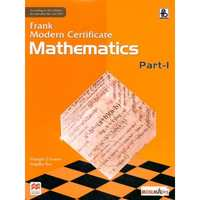## Chapter 16: Similarity

Exercise 16.1Exercise 16.2
Exercise 16.1

### Frank solutions for Class 9 Maths ICSE Chapter 16 Similarity Exercise 16.1

Exercise 16.1 | Q 1.1

In ΔABC, D and E are the mid-point on AB and AC such that DE || BC.
If AD = 4, AE = 8, DB = x - 4 and EC = 3x - 19, find x.

Exercise 16.1 | Q 1.2

In ΔABC, D and E are the mid-point on AB and AC such that DE || BC.
If AD : BD = 4 : 5 and EC = 2.5cm, find AE.

Exercise 16.1 | Q 1.3

In ΔABC, D and E are the mid-point on AB and AC such that DE || BC.
If AD = 4x - 3, AE = 8x - 7, BD = 3x - 1 and CE = 5x - 3,Find x.

Exercise 16.1 | Q 1.4

In ΔABC, D and E are the mid-point on AB and AC such that DE || BC.
If AD = 8cm, AB = 12cm and AE = 12cm, find CE.

Exercise 16.1 | Q 2.1

If ΔABC, D and E are points on AB and AC. Show that DE || BC for each of the following case or not:
AB = 5.6cm, AD = 1.4cm, AC = 7.2cm, and AE = 1.8cm

Exercise 16.1 | Q 2.2

If ΔABC, D and E are points on AB and AC. Show that DE || BC for each of the following case or not:
AB = 10.8cm, BD = 4.5cm, AC = 4.8cm, and AE = 2.8cm

Exercise 16.1 | Q 2.3

If ΔABC, D and E are points on AB and AC. Show that DE || BC for each of the following case or not:
AD = 5.7cm, BD = 9.5cm, AE = 3.3cm, and EC = 5.5cm

Exercise 16.1 | Q 3

In figure, PQ is parallel to BC, AP : AB = 2 : 7. If QC = 0 and BC = 21,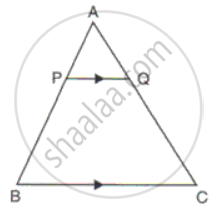Find
(i) AQ
(ii) PQ

Exercise 16.1 | Q 4

In ΔABC, DE is parallel to BC and DE = 3:8.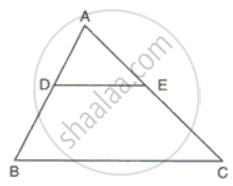Find:
(ii) AE, if AC = 16.

Exercise 16.1 | Q 5.1

In ΔABC, point D divides AB in the ratio 5:7, Find: "AE"/"EC"

Exercise 16.1 | Q 5.2

In ΔABC, point D divides AB in the ratio 5:7, Find: "AD"/"AB"

Exercise 16.1 | Q 5.3

In ΔABC, point D divides AB in the ratio 5:7, Find: "AE"/"AC"

Exercise 16.1 | Q 5.4

In ΔABC, point D divides AB in the ratio 5:7, Find: BC, If DE = 2.5cm

Exercise 16.1 | Q 5.5

In ΔABC, point D divides AB in the ratio 5:7, Find: DE, If BC = 4.8cm

Exercise 16.1 | Q 6

If ΔPQR, AB is drawn parallel to QR. If PQ = 9cm, PR = 6cm and PB = 4.cm, find the length of AP.

Exercise 16.1 | Q 7

In ΔABC, MN is drawn parallel to BC. If AB = 3.5cm, AM : AB = 5 : 7 and NC = 2cm, find:
(i) AM
(ii) AC

Exercise 16.1 | Q 8

The sides PQ and PR of the ΔPQR are produced to S and T respectively. ST is drawn parallel to QR and PQ: PS = 3:4. If PT = 9.6 cm, find PR. If 'p' be the length of the perpendicular from P to QR, find the length of the perpendicular from P to ST in terms of 'p'.

Exercise 16.1 | Q 9

ΔABC is right angled at A. AD is drawn perpendicular to BC. If AB = 8cm and AC = 6cm, calculate BD.

Exercise 16.1 | Q 10

In the figure, PR || SQ. If PR = 10cm, PT = 5cm, TQ = 6cm and ST = 9cm, calculate RT and SQ.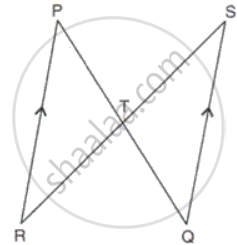Exercise 16.1 | Q 11

ABCD is a parallelogram whose sides AB and BC are 18cm and 12cm respectively. G is a point on AC such that CG : GA = 3 : 5 BG is produced to meet CD at Q and AD produced at P. Prove that ΔCGB ∼ ΔAGP. Hence, fi AP.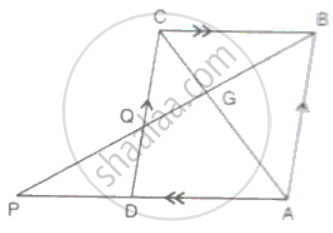Exercise 16.1 | Q 12

In ΔABC, BP and CQ are altitudes from B and C on AC and AB respectively. BP and CQ intersect at O. Prove that
(i) PC x OQ = QB x OP

(ii) "OC"^2/"OB"^2 = ("PC" xx "PO")/("QB" xx "QO")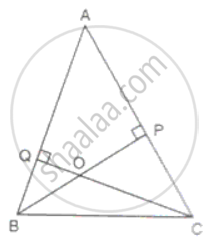Exercise 16.1 | Q 13

In the figure, PQR is a straight line and PS || RT. If QS = 12cm, QR = 15cm, QT = 10cm and RT = 6cm, find PQ and PS.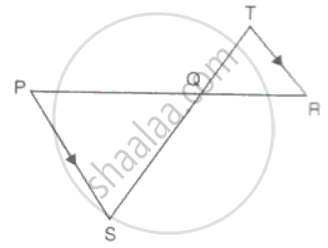Exercise 16.1 | Q 14

The diagonal AC of a parallelogram ABCD intersects DP at the point Q, where P is any point on side AB. Prove that CQ x PQ = QA x QD.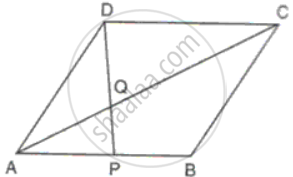Exercise 16.1 | Q 15

AM and DN are the altitudes of two similar triangles ABC and DEF. Prove that: AM : DN = AB : DE.

Exercise 16.1 | Q 16

Prove that the external bisector of an angle of a triangle divides the opposite side externally n the ratio of the sides containing the angle.

Exercise 16.1 | Q 17

In the figure, AB || RQ and BC || SQ, prove that "PC"/"PS" = "PA"/"PR".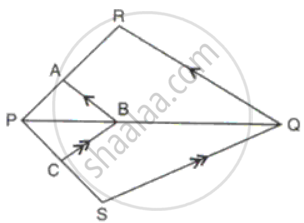Exercise 16.1 | Q 18

In the figure, DE || AC and DC || AP. Prove that "BE"/"EC" = "BC"/"CP"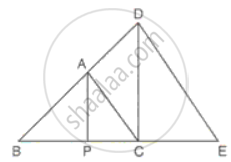Exercise 16.1 | Q 19

PQ is perpendicular to BA and BD is perpendicular to AP.PQ and BD intersect at R. Prove that ΔABD ∼ ΔAPQ and "AB"/"AP" = "BD"/"PQ".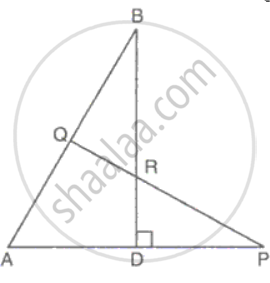Exercise 16.1 | Q 20

Through the vertex S of a parallelogram PQRS, a line is drawn to intersect the sides Qp and QR produced at M and N respectively. Prove that "SP"/"PM" = "MQ"/"QN" = "MR"/"SR"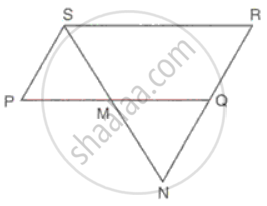Exercise 16.1 | Q 21.1

In a quadrilateral PQRS, the diagonals PR and QS intersect each other at the point T. If PT:TR = QT :TS = 1:2, show that ΔPTQ - DRTS

Exercise 16.1 | Q 21.2

In a quadrilateral PQRS, the diagonals PR and QS intersect each other at the point T. If PT:TR = QT :TS = 1:2, show that TP:TQ = TR:TS

Exercise 16.1 | Q 22

In the given figure, PB is the bisector of ABC and ABC =ACB. Prove that:
a. BC x AP = PC x AB
b. AB:AC = BP: BC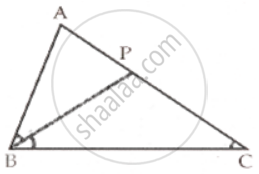Exercise 16.1 | Q 23

In a right-angled triangle ABC, ∠B = 90°, P and Q are the points on the sides AB and AC such as PQBC, AB = 8 cm, AQ = 6 cm and PA:AB = 1:3. Find the lengths of AC and BC.

Exercise 16.2

### Frank solutions for Class 9 Maths ICSE Chapter 16 Similarity Exercise 16.2

Exercise 16.2 | Q 1

Given that ΔABC ∼ ΔPRQ, name the corresponding angles and the corresponding sides.

Exercise 16.2 | Q 2

In ΔABC, DE || BC such that AD =1.5 cm, DB = 3 cm and AE = 1 cm. Find AC.

Exercise 16.2 | Q 3

Given is a triangle with sides 3 cm, 5 cm and 6 cm. Find the sides of a triangle which is similar to the given triangle and its shortest side is 4.5 cm.

Exercise 16.2 | Q 4

Two figures are similar. If the ratio of their perimeters is 8:16. What will be the ratio of the corresponding sides?

Exercise 16.2 | Q 5

Harmeet is 6 feet tall and casts a shadow of 3 feet long. What is the height of a nearby pole if it casts a shadow of 12 feet long at the same time?

Exercise 16.2 | Q 6

The areas of two similar triangles are 16cm2 and 9cm2 respectively. If the altitude of the smaller triangle is 1.8cm, find the length of the altitude corresponding to the larger triangle.

Exercise 16.2 | Q 7

The areas of two similar triangles are 169cm2 and 121cm2 respectively. If one side of the larger triangle is 26cm, find the length of the corresponding side of the smaller triangle.

Exercise 16.2 | Q 8

D and E are points on the sides AB and AC  of ΔABC such that DE | | BC and divides ΔABC into two parts, equal in area. Find "BD"/"AB".

Exercise 16.2 | Q 9

In ΔABC, DE is drawn parallel to BC cutting AB in the ratio 2 : 3. Calculate:
(i) ("area"(Δ"ADE"))/("area"(Δ"ABC")

(i) ("area"("trapeziumEDBC"))/("area"(Δ"ABC"))

Exercise 16.2 | Q 10.1

In ΔABC, AB = 8cm, AC = 10cm and ∠B = 90°. P and Q are the points on the sides AB and AC respectively such that PQ = 3cm ad ∠PQA = 90. Find: The area of ΔAQP.

Exercise 16.2 | Q 10.2

In ΔABC, AB = 8cm, AC = 10cm and ∠B = 90°. P and Q are the points on the sides AB and AC respectively such that PQ = 3cm ad ∠PQA = 90. Find: Area of quadrilateral PBCQ: area of ΔABC.

Exercise 16.2 | Q 11.1

Find the scale factor in each of the following and state the type of size transformation:
Image length = 6cm, Actual length = 4cm.

Exercise 16.2 | Q 11.2

Find the scale factor in each of the following and state the type of size transformation:
Actual length = 12cm, Image length = 15cm.

Exercise 16.2 | Q 11.3

Find the scale factor in each of the following and state the type of size transformation:
Image length = 8cm, Actual length = 20cm.

Exercise 16.2 | Q 11.4

Find the scale factor in each of the following and state the type of size transformation:
Actual area = 64m2, Model area = 100cm2

Exercise 16.2 | Q 11.5

Find the scale factor in each of the following and state the type of size transformation:
Model area = 75cm2, Actual area = 3cm2

Exercise 16.2 | Q 11.6

Find the scale factor in each of the following and state the type of size transformation:
Model volume = 200cm3, Actual volume = 8cm3

Exercise 16.2 | Q 12.1

ΔABC has been reduced by a scale factor 0.6 to ΔA'B'C'/ Calculate:Length of B' C', if BC = 8cm

Exercise 16.2 | Q 12.2

ΔABC has been reduced by a scale factor 0.6 to ΔA'B'C'/ Calculate: Length of AB, if A'B' = 5.4cm

Exercise 16.2 | Q 13.1

ΔABC is enlarged, with a scale factor 5. Find: A'B', if AB = 4cm

Exercise 16.2 | Q 13.2

ΔABC is enlarged, with a scale factor 5. Find: BC, f B'C' = 16cm

Exercise 16.2 | Q 14

ΔXYZ is enlarged to ΔX'Y'Z'. If XY = 12cm, YZ = 8cm and XZ = 14cm and the smallest side of ΔX'Y'Z' is 12cm, find the scale factor and use it to find the length of the other sides of the image ΔX'Y'Z'.

Exercise 16.2 | Q 15

On a map drawn to a scale of 1: 2,50,000, a triangular plot of land has the following measurements:
AB = 3 cm, BC = 4 cm, ∠ABC = 90°. Calculate:
(i) The actual length of AB in km.
(ii) The area of Plot in sq. km.

Exercise 16.2 | Q 16

The dimensions of the model of a building are 1.2m x 75cm x 2m. If the scale factor is 1 : 20; find the actual dimensions of the building.

Exercise 16.2 | Q 17.1

The scale of a map is 1 : 50000. The area of a city is 40 sq km which is to be represented on the map. Find: The area of land represented on the map.

Exercise 16.2 | Q 17.2

The scale of a map is 1 : 50000. The area of a city is 40 sq km which is to be represented on the map. Find: The length of a scale in km represented by 1cm on the map.

Exercise 16.2 | Q 18.1

A plot of land of area 20km2 is represented on the map with a scale factor of 1:200000. Find: The number of KM represented by 2cm on the map.

Exercise 16.2 | Q 18.2

A plot of land of area 20km2 is represented on the map with a scale factor of 1:200000. Find: The ground area in km2 that is represented by 2cm2 on the map.

Exercise 16.2 | Q 18.3

A plot of land of area 20km2 is represented on the map with a scale factor of 1:200000. Find: The area on the map that represented the plot of land.

Exercise 16.2 | Q 19.1

A map is drawn to scale of 1:20000. Find: The distance covered by 6cm on the map

Exercise 16.2 | Q 19.2

A map is drawn to scale of 1:20000. Find: The distance on the map representing 4km

Exercise 16.2 | Q 19.3

A map is drawn to scale of 1:20000. Find: The area of the lake on the map which has an actual area of 12km2

Exercise 16.2 | Q 20.1

A model of cargo tuck is made to a scale of 1:40. The length of the model is 15cm. Calculate: The length of the truck

Exercise 16.2 | Q 20.2

A model of cargo tuck is made to a scale of 1:40. The length of the model is 15cm. Calculate: The volume of the model if the volume of the truck is 6m3

Exercise 16.2 | Q 20.3

A model of cargo tuck is made to a scale of 1:40. The length of the model is 15cm. Calculate: The base area of the truck, if the base area of the model is 30m2

Exercise 16.2 | Q 21.1

A model of a ship is made to a scale of 1:500. Find: The length of the ship, if length of the model is 1.2.

Exercise 16.2 | Q 21.2

A model of a ship is made to a scale of 1:500. Find: The area other deck o the ship, if the area of the deck of its model is m2

Exercise 16.2 | Q 21.3

A model of a ship is made to a scale of 1:500. Find: The volume of the model when the volume of the ship is 1km

Exercise 16.2 | Q 22.1

On a map drawn to a scale of 1:25000, a rectangular plot of land has sides 12cm x 16cm. Calculate: The diagonal distance of the plot in km

Exercise 16.2 | Q 22.2

On a map drawn to a scale of 1:25000, a rectangular plot of land has sides 12cm x 16cm. Calculate: The area of the plot in sq km

Exercise 16.2 | Q 23.1

On a map drawn to a scale of 1:25000, a triangular plot of land is right angled and the sides forming the right angle measure 225cm and 64cm.Find: The actual length of the sides in km

Exercise 16.2 | Q 23.2

On a map drawn to a scale of 1:25000, a triangular plot of land is right angled and the sides forming the right angle measure 225cm and 64cm.Find: The area of the plot in sq. km.

Exercise 16.2 | Q 24

In a triangle ABC, AB = 4 cm, BC = 4.5 cm and CA = 5 cm. Construct ΔABC. Find the image A'B'C of the ΔABC obtained by enlarging it by a scale factor 2. Measure the sides of the image A'B'C' and show that AB:A'B' = AC:B'C' = CA:C'A'

## Chapter 16: Similarity

Exercise 16.1Exercise 16.2## Frank solutions for Class 9 Maths ICSE chapter 16 - Similarity

Frank solutions for Class 9 Maths ICSE chapter 16 (Similarity) include all questions with solution and detail explanation. This will clear students doubts about any question and improve application skills while preparing for board exams. The detailed, step-by-step solutions will help you understand the concepts better and clear your confusions, if any. Shaalaa.com has the CISCE Class 9 Maths ICSE solutions in a manner that help students grasp basic concepts better and faster.

Further, we at Shaalaa.com provide such solutions so that students can prepare for written exams. Frank textbook solutions can be a core help for self-study and acts as a perfect self-help guidance for students.

Concepts covered in Class 9 Maths ICSE chapter 16 Similarity are Figures Between the Same Parallels, Triangles with the Same Vertex and Bases Along the Same Line, Concept of Area, Similarity.

Using Frank Class 9 solutions Similarity exercise by students are an easy way to prepare for the exams, as they involve solutions arranged chapter-wise also page wise. The questions involved in Frank Solutions are important questions that can be asked in the final exam. Maximum students of CISCE Class 9 prefer Frank Textbook Solutions to score more in exam.

Get the free view of chapter 16 Similarity Class 9 extra questions for Class 9 Maths ICSE and can use Shaalaa.com to keep it handy for your exam preparation